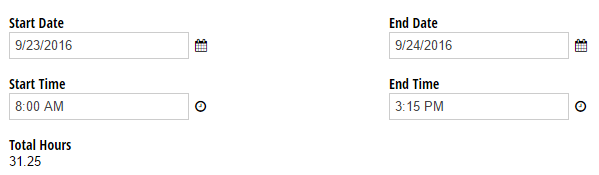# How can I calculate total hours based on Date/Time fields?

To calculate the number of hours based on the difference between two Date fields:

1. Add two Date fields to your form, labelled “Start Date” and “End Date”.
2. Add two Time fields to your form, labelled “Start Time” and “End Time”.
3. Add a Calculation field labelled Total Hours, and insert the following expression: `=(Math.Round((EndDate.AddMinutes(EndTime.Minute + (EndTime.Hour * 60)) - StartDate.AddMinutes(StartTime.Minute + (StartTime.Hour * 60))).TotalMinutes / 15) / 4)`
4. Now, when the user inputs their start and end times, the Calculation field will automatically display the total number of hours in 15 minute increments: